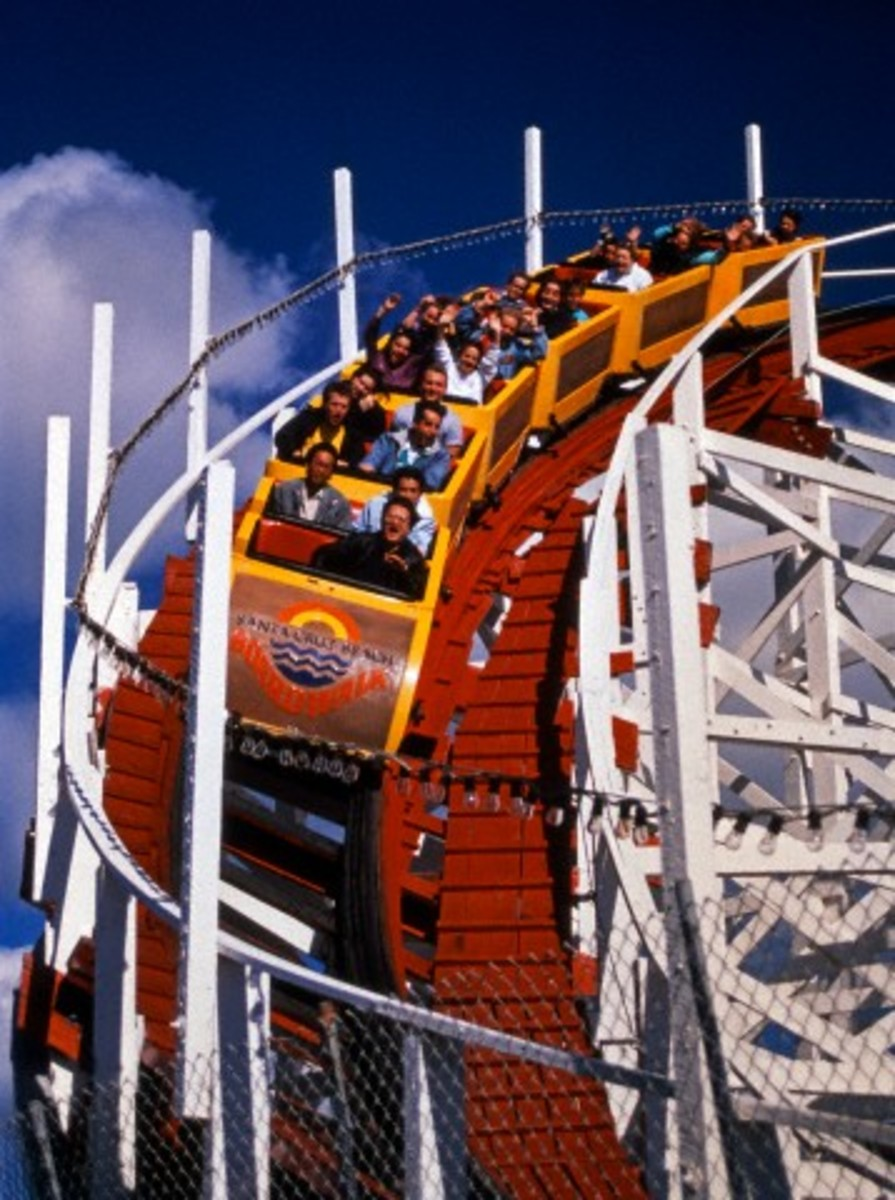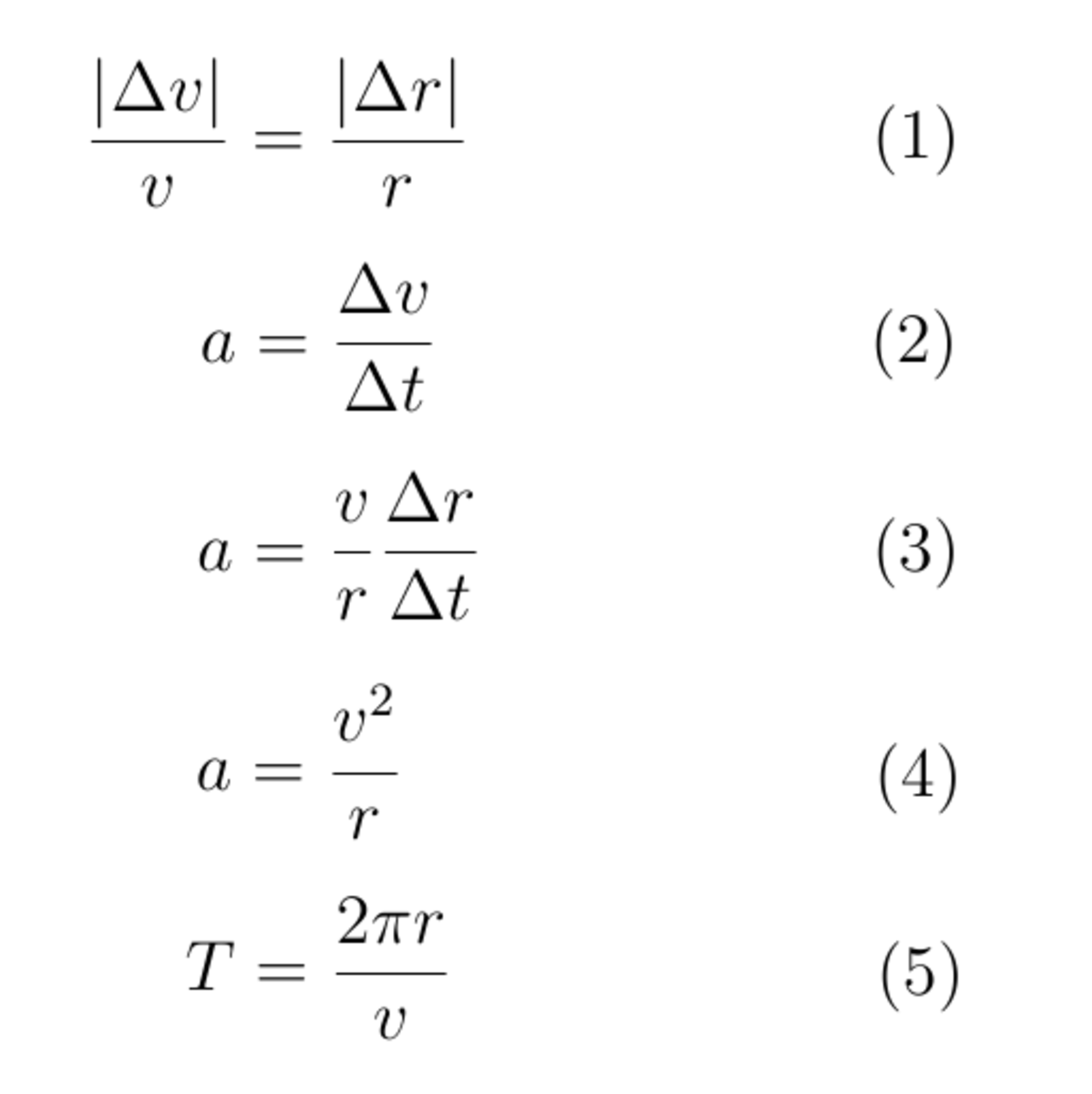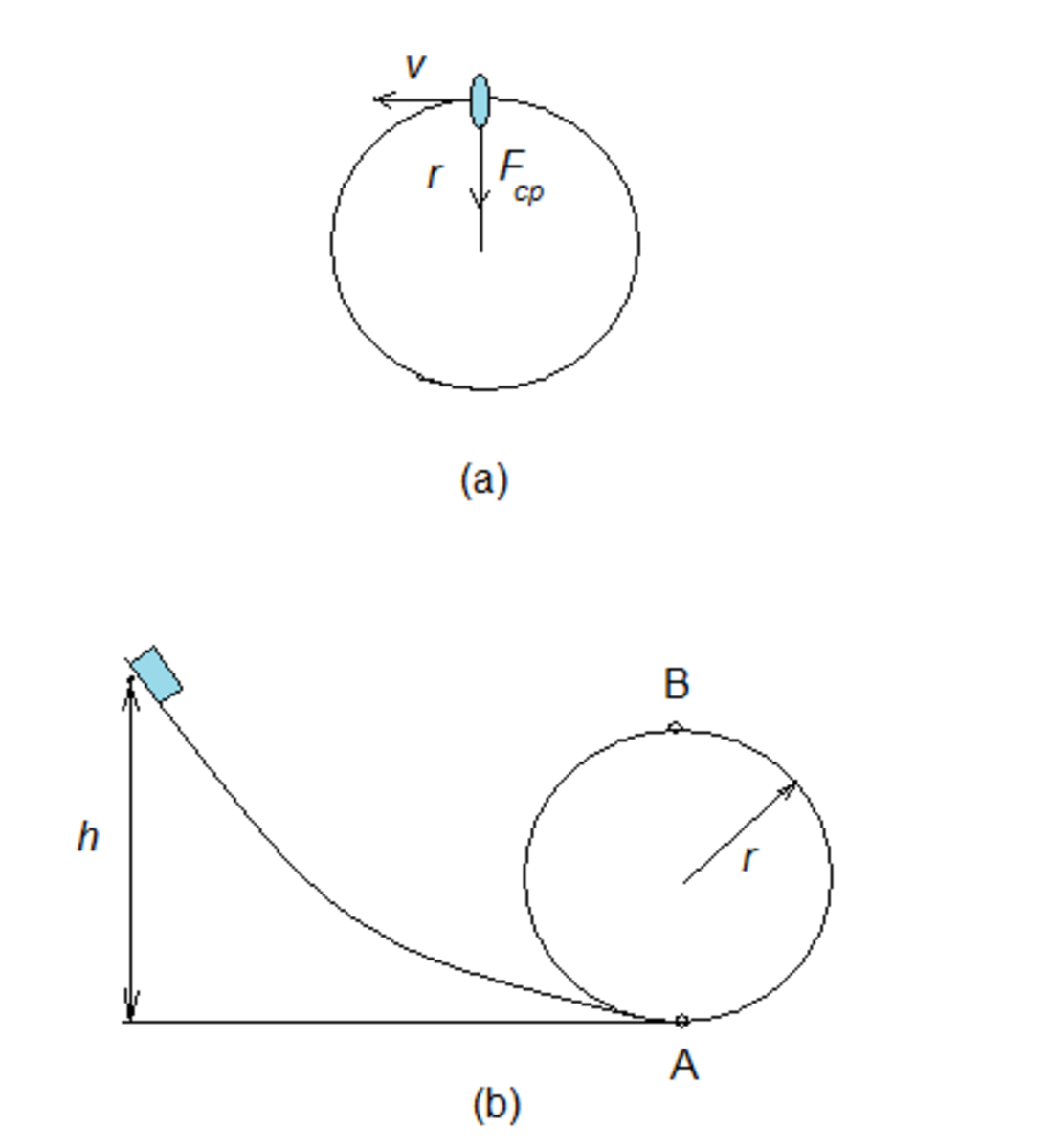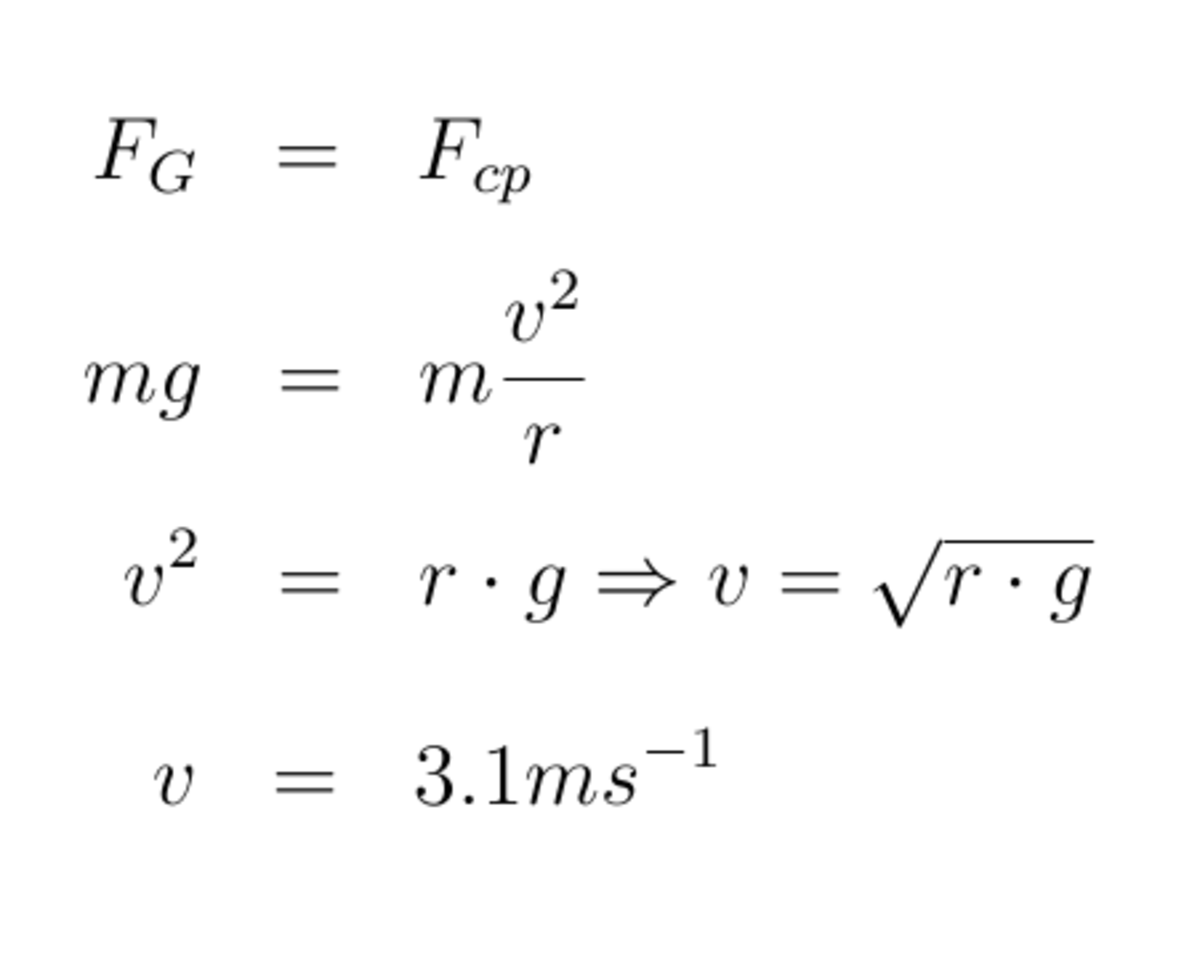# How does a roller coaster work? - the physics of circular motion

• Author:
• Updated date:

Have you ever been to an amusement park? Might you had a roller coaster trip, even one with a loop. Indeed, how come that in one moment we are completely upside down but stable. Of course, we are bound in a roller coaster. However, in an equivalent situation bodies would not have fallen even if were not bound. For example, imagine a bucket of water on the rope that one rotate vertically to the ground. If the rotation is fast enough, water will not spill.

While situation like this with rotating water could be appreciated as experiments, circular motion is very present in our everyday life. Driving a car at a roundabout, laundry and carousel trip are some of instances of the circular motion. By our experience we know on what we have to take attention in these situation. We have to slow the car on the curve, we use centrifugal force to wash clothes. But, what are the physical rules underline these situations.

## Uniform circular motion

Note that in every mentioned example a force act on a rotating body toward the centre of rotation. Within the car it is the friction, and because of that the more slippery road the speed should be smaller. Within rotating bucket it is tension in the rope. So, the circular motion is caused by a force towards the centre of rotation and this force is called the centripetal force.

This observation is fully in line with the Newton's laws of motion. According to the first one law, a body can either stay at rest or move uniformly along a line. Since circular motion is non of these two, there must be a net force to the body.Fig. 1. Would you like to take a roller coaster trip? :-)

Imagine now that experiment with the bucket of water we performe on a way that plane of motion will be parallel with the ground.

Assume that the rope is cut in one moment. Looking from above, bucket will continue motion along a tangent with constant speed.

At least the bucket will fall to the graund but free fall is here independent motion; if there is no gravitational field the bucket would move uniformly straight forward.

Thus, we conclude that a force maintaining circular motion must act towards the centre of rotation. In this case the centripetal force is tension in the rope.

## Acceleration of the uniform circular motion

It is clear that if centripetal force acts towards the centre, than there is aslo acceleration in the same direction, and opposite. Analizing the geometry of the motion we will now see that a particle moving uniformly along a circle accelerates towards the centre.

The geometry of a circular motion is seen in Figure 2. Let in the moment t1 the particle be in the point A, whereas in the moment t2 it is in the point B. Translating velocity vector we obtained a triangle as Figure 2 (b) showes. It is easy to see that this triangle is similar to the triangle that is formed by the two position vectors. Thus, it holds equation (1), where v is the length of velocity vector v1 i.e. v2, and r is the length of position vector r1 i.e. r2. Now, combining formulas (1) and (2) we have ralation (3). Having in mind that positios A and B are extremely close to each other, we conclude that when t2-t1 approaches zero, the ratio in relation (3) approaches the speed v. This leads to the relation (4). This relation shows that in uniform circular motion, the acceleration is directed toward the centre of the circle and its magnitude is v2/r.Fig. 3. Two basic relation of the uniform circular motion are (4) and (5).

## An experiment

We can illustrate the derived formula for centripetal acceleration with a simple experiment. Applying the second Newton's law of motion it follows Fcp= m x acp, where m is the mass of moving particle. Imagine a small ball fixed at the end of rope rotating with only one hand. As a handle can serve some old pen while the weight on the opposite end of rope can be keys (if nothing more suitable).

First of all, note that once having initial speed the system will rotate theoretically without any movements of our hand. Of course our little effort is necessary to maintain circular motion but this is because of friction between the handle and the rope and influence of atmosphere. If we could ignore all external influences, the tension of rope will be enough to preserve once established circular motion. (In such “home made” experiment we can hardly avoid external influences, but for example the only force preserving a moon orbit around a planet is the gravitational interaction. Gravitational interaction in that case is a centripetal force.)

The basis parameters of the system are the mass of a ball, the radius of motion, the velocity of motion and the value of centripetal force – that is equal to the weight of keys. Let now assume that in the experiment the weight is doubled, while the mass of ball and radius remain the same. How the speed of ball will change? Intuitively we know that it will increase. With a little skill and suitable equipment the speed can be measured by counting circles in a certain time frame. We can convinced ourselves that the sped will increase appoximately 1.4 times (which is square root of 2). This confirm the relation (4) i.e. the relation

Scroll to Continue

Fcp = m * v2 / r.

## Period of the uniform circular motion

In addition to relation (4), another important equation for the circular motion is those one connecting the period, radius and radius of motion.

Having in mind the basic equation for velocity (v=s/t) and the equation of circumference (s=2rπ) is obvious that the relation (5) holds.

The parameter T denotes the period of motion; and as is known it is inversely proportional to the frequency of motion f, T = 1 / f.

## Russian or American Mountains!? :-)

The forerunners of present-day roller coasters has been dated at about 1600. Those the oldest roller coasters come from Russia, especially from St Petersburg and surroundings. Actually, it was specially designed ice hills reaching height of 20 and more meters. That new fun, known as "Russian Mountains" started to spread to Europe.

Interestingly, nowadays the term "Russian Mountains" for a roller coaster is preserved in most Latin languages while the Russian term is "American Mountains".

## Centrifugal force

As is well known to the first Newton's law of motion, bodies are inert. This means if a body stay at rest it tend to continue staying at rest; and if it move uniformly straight forward it tend to keep this motion. The centrifugal force is a result of body's inertia opposing to centripetal force. This two effects results with a body resistance against the centripetal force. The magnitude of centrifugal force is always the same as the centripetal one.

Washing machine is a nice example of usage of centrifugal force. Water droplets tend to maintain their direction of movement (and that is a tangent line), which is enabled by holes in the machine rotor.

When driving a roller coaster, in the moment when we are upside down we actually press up since the our speed. We don't fall because our weight is overcome by our inertia. Just as water droplets in the washing machine we tend to move away from the centre of rotation. The pressure on chairs, which is net result of two forces, depend on the roller coaster speed.

## Case studies

Rotating bucket of water

Let in the described experiment with bucket of water the length of rope be a metre. The plane of uniform circular motion is vertical to the ground. What is the frequency of the motion? According to the previous considerations, in order to maintain the motion the centripetal force should be at least as high as the weight of water. Thus, the speed should be at least 3.1 m/s as is calculated in the Figure 5. The related period now follows from equation (5), and the frequency is the inverse value of period. Since one circle takes around 2 second , the frequency is 0.5 Hz.

A cart at an incline

Imagine a cart freely rolling on a slope (Figure 4 b). Let the radius of loop be r. What is the hight h if the cart succcessfuly passes the loop? Firstly, initial potential energy is equal to the sum of potential and kinetic energy in the point B. It holds mgh = mg2r+mv2/2. Combining this with the expression for the speed (as in previous exercise) we have h=2r +r/2.Fig. 4. Rotating bucket of water and a cart at an incline - typical case studies for uniform circular motion.Fig. 5. Calculation of the speed of a rotating bucket of water.

A spacecraft in orbit

A spacecraft is in orbit at a height of 220km above the surface of the Earth. The period of its orbit is 88.7 min. What is the speed of a sattelite in its orbit? The spacecraft circles around the Earth with the rocker engine turned off.

Thus, here we have uniform circular motion with the gravitational force as a centripetal force. The speed, as well as the centripetal acceleration, easily can be calculated from the relations above. The radius of Earth is 6.37 x 106 m. The centripetal acceleration is 9.18 m/s2, slightly less than the gravitational acceleration on the Earth surface. Note that this is the value of gravitational acceleration at the hight of 220 km. This result is expected since the spacecraft is actually falling radially toward the Earth. However, due to the initial speed of the craft the gravitational acceleration will cause circular motion (instead of free fall through a line).

## A short quiz at the end :-)

For each question, choose the best answer. The answer key is below.

1. If the speed increases twice and the other parameters stay unchanged, the value of radius will...
• increases twice
• increases 4 times
• decline twice
• remains the same
2. Which statement is false ? A body in the uniform circular motion...
• uniformly accelerates towards the centre
• uniformly move along a tangent line
• accelerates along a tangent line
3. What force keeps the car in a curve?
• gravity
• friction
• tension
• inertia

1. increases 4 times
2. accelerates along a tangent line
3. friction

## Summary

• Uniform circular motion is a complex motion composed by the uniform linear motion along a tangent line and the uniformly accelerated motion towards the centre.
• Centripetal force is the name of the force causing circular motion. Depending on a given situation, it can be gravitational interaction,friction, tension in the rope etc.
• Centrifugal force is the result of body's inertia and the centripetal force.
• Two basic formulas related to the uniform circular motion are (4) and (5).

Bianca on August 12, 2017:

Thank you so much for this! It was really helpful and was easily understood.

Nic on December 02, 2013:

i can't even believe my eyes

Sam on December 02, 2013:

i know who you really are

Mike on December 02, 2013:

u suk

Sam on December 02, 2013:

what's going on?